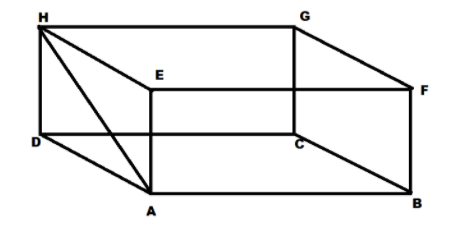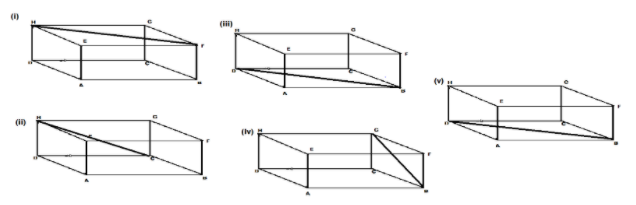QuestionAnswers

# State Yes or No. You can draw line segments on the surface of the face of a cuboid.Verified
129.3k+ views
Hint: Here we will check if we can draw a line segment on the surface of the cuboid or not and then decide whether the given statement is true or false.
A line segment is a line joining two fixed points.
Also a line segment can be drawn on a flat surface

We know that a line segment can be drawn on a flat surface but on curved surfaces.
And also, a cuboid is a flat surface.
This implies that the surface of the face of the cuboid is flat.
Therefore a line segment can be drawn on the surface of the face of a cuboid.

The line segment on the surface of a face of cuboid can be drawn as follows:-Hence, the given statement “You can draw line segments on the surface of the face of a cuboid.” is true.
Hence we can state yes for the given statement.

Note: Students should note that cuboid has six faces and every face has a flat surface.

Therefore, a line segment can be drawn on any of the six faces of the cuboid.

Hence the figure can also be drawn in following ways: